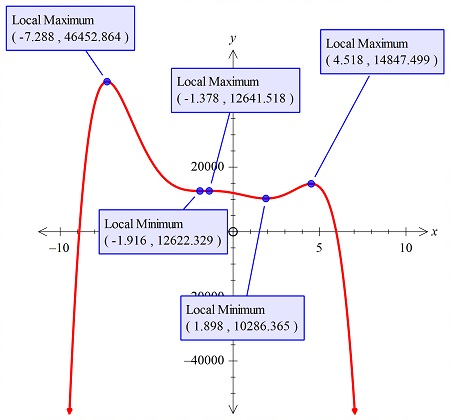# Graph the function f (x) = -x^6 - 5 x^5 + 49 x^4 + 122 x^3 - 315 x^2 - 900 x + 12,000. Then...

## Question:

Graph the function {eq}\displaystyle f (x) = -x^6 - 5 x^5 + 49 x^4 + 122 x^3 - 315 x^2 - 900 x + 12,000 {/eq}. Then estimate any relative extrema.

## Graphs:

When in the question if it is asked to plot the curve and estimate the relative extremes, then we use the same plot where the curve is shown, to plot the extreme points.

The graph of the function is shown below:The relative extremas are also shown.